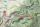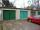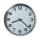# Two cyclists

Two cyclists started from crossing in the same time. One goes to the north speed 20 km/h, the second eastward at speed 26 km/h. What will be the direct distance cycling 30 minutes from the start?

Result

s =  16.401 km

#### Solution:Leave us a comment of example and its solution (i.e. if it is still somewhat unclear...):

Showing 0 comments:Be the first to comment!#### To solve this example are needed these knowledge from mathematics:

Pythagorean theorem is the base for the right triangle calculator. Try conversion angle units angle degrees, minutes, seconds, radians, grads.

## Next similar examples:

1. Cross roadFrom the junction of two streets that are perpendicular to each other, two cyclists (each on another street) walked out. One ran 18 km/h and the second 24 km/h. How are they away from a) 6 minutes, b) 15 minutes?
2. Two aircraftFrom the airport will start simultaneously two planes, which fly tracks are perpendicular to each other. The first flying speed of 680 km/h and the second 840 km/h. Calculate how far the aircraft will fly for half an hour.
3. A trip to the peak DryCalculate what percentage is rising trip from Strečno to peak Suchý (Dry peak) longer than the descent back. Team trio of tourists (Palo, Peter and Andrew) rise took 4:21 and descent 3:08.
4. StormSo far, a storm has traveled 35 miles in 1/2 hour in direction straight to observer. If it is currently 5:00 p. m. and the storm is 105 miles away from you, at what time will the storm reach you? Explain how you solved the problem.
5. A truckA truck departs from a distribution center. From there, it goes 20km west, 30km north and 10km west and reaches a shop. How can the truck reach back to the distribution center from the shop (what is the shortest path)?
6. Holidays - on poolChildren's tickets to the swimming pool stands x € for an adult is € 2 more expensive. There was m children in the swimming pool and adults three times less. How many euros make treasurer for pool entry?
7. Rectangular triangle PQRIn the rectangular triangle PQR, the PQ leg is divided by the X point into two segments of which longer is 25cm long. The second leg PR has a length 16 cm. The length of the RX is 20 cm. Calculate the length p of side RQ. The result is round to 2 decimal
8. ChordIt is given to a circle k(r=6 cm) and the points A, B such that / AB / = 8 cm lies on k. Calculate the distance of the center of circle S to the midpoint C of the segment AB.
9. LathsThere are two laths in the garage opposite one another: one 2 meters long and the other 3 meters long. They fall against each other and lean against the opposite walls of the garage both laths and touch at a height of 70 cm above the garage floor. How wide
10. Broken treeThe tree was 35 meters high. The tree broke at a height of 10 m above the ground. Top but does not fall off it refuted on the ground. How far from the base of the tree lay its peak?
11. WindbreakA tree at a height of 3 meters broke in the windbreak. Its peak fell 4.5 m from the tree. How tall was the tree?
12. HelmetIn nowadays cycling better results could be obtained not only due to rider physical preparation but also thanks to using updated technologies. One of the most important part of rider´s equipment is his or her helmet. While most bikers wear a helmet mainly
13. ClockHow many hours are 15 days?
14. The ditchDitch with cross section of an isosceles trapezoid with bases 2m 6m are deep 1.5m. How long is the slope of the ditch?
15. Reference angleFind the reference angle of each angle:
16. SatinSanusha buys a piece of satin 2.4 m wide. The diagonal length of the fabric is 4m. What is the length of the piece of satin?
17. If theIf the tangent of an angle of a right angled triangle is 0.8. Then its longest side is. .. .